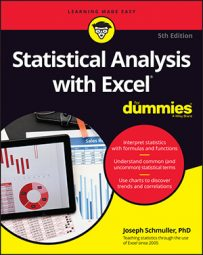##### Statistical Analysis with Excel For DummiesExcel offers a wide range of statistical functions you can use to calculate a single value or an array of values in your Excel worksheets. The Excel Analysis Toolpak is an add-in that provides even more statistical analysis tools.

## Some Excel worksheet functions for statistical analysis

Excel can help you make all sorts of calculations. Here’s a selection of Excel’s statistical worksheet functions. Each one returns a value into a selected cell.

Check out these functions for central tendency and variability:

 Function What it calculates AVERAGE Mean of a set of numbers AVERAGEIF Mean of a set of numbers that meet a condition AVERAGEIFS Mean of a set of numbers that meet one or more conditions HARMEAN Harmonic mean of a set of positive numbers GEOMEAN Geometric mean of a set of positive numbers MODE.SNGL Mode of a set of numbers MEDIAN Median of a set of numbers VAR.P Variance of a set of numbers considered to be a population VAR.S Variance of a set of numbers considered to be a sample STDEV.P Standard deviation of a set of numbers considered to be a population STDEV.S Standard deviation of a set of numbers considered to be a sample STANDARDIZE A standard score based on a given mean and standard deviation

These handy functions for relative standing can also be very useful:

 Function What it calculates RANK.EQ Rank of a number in a set of numbers. If more than one number has the same rank, it returns the top rank of those numbers. RANK.AVG Rank of a number in a set of numbers. If more than one number has the same rank, it returns their average. PERCENTRANK.INC Rank of a number in a set of numbers, expressed as a percent of the numbers it’s greater than or equal to. PERCENTRANT.EXC Rank of a number in a set of numbers, expressed as a percent of the numbers it’s greater than. PERCENTILE.INC The indicated percentile in a set of numbers, in terms of “greater than or equal to.” PERCENTILE.EXC The indicated percentile in a set of numbers, in terms of “greater than.” QUARTILE.INC The 1st, 2nd, 3rd, or 4th quartile of a set of numbers, in terms of “greater than or equal to.” QUARTILE.EXC The 1st, 2nd, 3rd, or 4th quartile of a set of numbers, in terms of “greater than.”

These functions for correlation and regression are also good ones to know:

 Function What it Calculates CORREL Correlation coefficient between two sets of numbers PEARSON Same as CORREL. (Go figure!) RSQ Coefficient of determination between two sets of numbers (square of the correlation coefficient) SLOPE Slope of a regression line through two sets of numbers INTERCEPT Intercept of a regression line through two sets of numbers STEYX Standard error of estimate for a regression line through two sets of numbers

## Excel array functions for statistical analysis

An array formula calculates a set of values rather than just one. Here are Excel’s statistical array functions. Each one returns an array of values into a selected array of cells.

In the Microsoft 365 version of Excel, you select one cell, enter the formula, press Enter, and the remaining values spill into the remaining cells of the array.

 Function Calculates An Array Of … FREQUENCY Frequencies of values in a set of values MODE.MULT Modes of a set of numbers LINEST Regression statistics based on linear regression through two or more sets of numbers LOGEST Regression statistics based on curvilinear regression through two or more sets of numbers TREND Numbers in a linear trend, based on known data points GROWTH Numbers in a curvilinear trend, based on known data points

## Excel data analysis tools

Excel’s Analysis ToolPak is a helpful add-in that provides an extensive set of statistical analysis tools. Here are some of the tools in the ToolPak. Note that the final tool, Logistic Regression, is in XLMiner’s Analysis ToolPak, not Excel’s.

 Tool What it Does Anova: Single Factor Analysis of variance for two or more samples Anova: Two Factor with Replication Analysis of variance with two independent variables, and multiple observations in each combination of the levels of the variables. Anova: Two Factor without Replication Analysis of variance with two independent variables, and one observation in each combination of the levels of the variables. You can use it for Repeated Measures ANOVA. Correlation With more than two measurements on a sample of individuals, calculates a matrix of correlation coefficients for all possible pairs of the measurements Covariance With more than two measurements on a sample of individuals, calculates a matrix of covariances for all possible pairs of the measurements Descriptive Statistics Generates a report of central tendency, variability, and other characteristics of values in the selected range of cells Exponential Smoothing In a sequence of values, calculates a prediction based on a preceding set of values, and on a prior prediction for those values F-Test Two Sample for Variances Performs an F-test to compare two variances Histogram Tabulates individual and cumulative frequencies for values in the selected range of cells Moving Average In a sequence of values, calculates a prediction which is the average of a specified number of preceding values Random Number Generation Provides a specified amount of random numbers generated from one of seven possible distributions Rank and Percentile Creates a table that shows the ordinal rank and the percentage rank of each value in a set of values Regression Creates a report of the regression statistics based on linear regression through a set of data containing one dependent variable and one or more independent variables Sampling Creates a sample from the values in a specified range of cells

Logistic Regression (in the XLMiner Analysis ToolPak, not Excel’s Analysis ToolPak) creates a report of regression statistics based on logistic regression through a set of data consisting of a quantitative independent variable and a dependent variable whose values can only be 0 or 1.